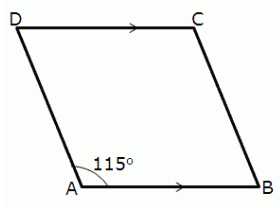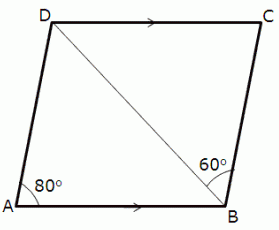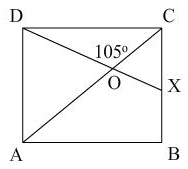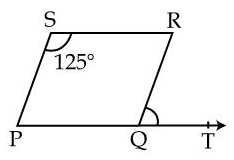# Test: Parallelograms Basic Properties

## 15 Questions MCQ Test Mathematics (Maths) Class 9 | Test: Parallelograms Basic Properties

Description
This mock test of Test: Parallelograms Basic Properties for Class 9 helps you for every Class 9 entrance exam. This contains 15 Multiple Choice Questions for Class 9 Test: Parallelograms Basic Properties (mcq) to study with solutions a complete question bank. The solved questions answers in this Test: Parallelograms Basic Properties quiz give you a good mix of easy questions and tough questions. Class 9 students definitely take this Test: Parallelograms Basic Properties exercise for a better result in the exam. You can find other Test: Parallelograms Basic Properties extra questions, long questions & short questions for Class 9 on EduRev as well by searching above.
QUESTION: 1

### In a parallelogram ABCD, if ∠A = 115°, then ∠B, ∠C and ∠D areSolution:

In a parallelogram ABCD, ∠D = 115° (Given)

Since, ∠A and ∠D are adjacent angles of parallelogram.

We know, Adjacent angles of a parallelogram are supplementary.

∠A + ∠D = 180°

∠A = 180° – 115°  = 65°

Measure of ∠A is 65°.

QUESTION: 2

Solution:
QUESTION: 3

### Which of the following is not a parallelogram?

Solution:
QUESTION: 4

In the figure ABCD is a ||gm in which ∠DAB = 80° and ∠DBC = 60°, then ∠CDB and ∠ADB are equal toSolution:
QUESTION: 5

A quadrilateral is a ______ if a pair of opposite sides is equal and parallel

Solution: Parallelogram because it have pair of opposite sides are equal and parallel
QUESTION: 6

Solution:
QUESTION: 7

In a parallelogram ABCD, if ∠A = 60°, then ∠D is equal to:

Solution:
QUESTION: 8

Each diagonal of a parallelogram divides it into two

Solution:
QUESTION: 9

Statement 2: AB = DC and AD=BCFor a quadrilateral ABCD to be a parallelogram,__________

Solution:
QUESTION: 10

Perimeter of a parallelogram is 22 cm. If the longer side, measures 6.5 cm, the measure of the shorter side will be:​

Solution:
Let the length of the shorter side be x cm
length of longer side= 6.5 cm
perimeter= 22 cm
>2[longer side+shorter side]=22
>2[6.5+x] = 22 = 22/2 =11
>6.5 + x =11 = 11 - 6.5 = 4.5
> x= 4.5
QUESTION: 11

In the figure ABCD is a square. A line segment DX cuts the side BC at X and the diagonal AC at O such that ∠COD = 105° and ∠OXC = y. Then y is equal toSolution:
Given :In the figure ABCD is a square. A line segment DX cuts the side BC at X and the diagonal AC at O such that COD = 105 degree and OXC = y
To find :y
Since DX is a line segment, then
angle COD + angle COX = 180 degrees {Angle sum property}
=> angle COX = 180 -105 = 75 degrees..(1)
Since ABCD is a square and AC is a diagonal , therefore all the angles in a square is 90 degree each and AC bisects the angle in half. {property}
=> angle ACB {  also OCX } = 90 /2 =45 degrees...(2)
In traingle COX
=> angle OCX + angle COX  + y = 180 degree { sum of angles in a triangle is 180 degree}
=> y = 180 - (45+75) {using eq 1 and 2}
=> y = 60 degrees Answer
QUESTION: 12

Which of the following is not true for a parallelogram?

Solution:
QUESTION: 13

In a parallelogram, if ∠A = (2x +25)° and ∠B = (3x – 5)°, the value of x is

Solution:
QUESTION: 14

If an angle of a parallelogram is four-fifths of the adjacent angle, then the angles of the parallelogram are​

Solution:
QUESTION: 15

In fig., PQRS is a parallelogram in which ∠PSR = 125°, ∠RQT is equal toSolution: